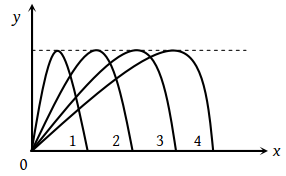Figure shows four paths for a kicked football. Ignoring the effects of air on the flight, rank the paths according to initial horizontal velocity component, highest first(1) 1, 2, 3, 4

(2) 2, 3, 4, 1

(3) 3, 4, 1, 2

(4) 4, 3, 2, 1

Concept Videos :-

#11-Projectile-Motion-1
#12-Projectile-Examples
#13-Projectile-Motion-2
#14-Projectile-Examples-1
#15-Projectile-Examples-2
#16-Solved-Examples-3
#17-Projectile-Examples-4
#18-Maximum-Height
#20-Derivation-of-Equation-of-Trajectory
#21-Solved-Examples-11

Concept Questions :-

Projectile motion

(4) $R=\frac{{u}^{2}\mathrm{sin}2\theta }{g}=\frac{2{u}_{x}{v}_{y}}{g}$

∴ Range ∝ horizontal initial velocity (ux

In path 4 range is maximum so football possess maximum horizontal velocity in this path.

Difficulty Level:

• 17%
• 7%
• 6%
• 72%